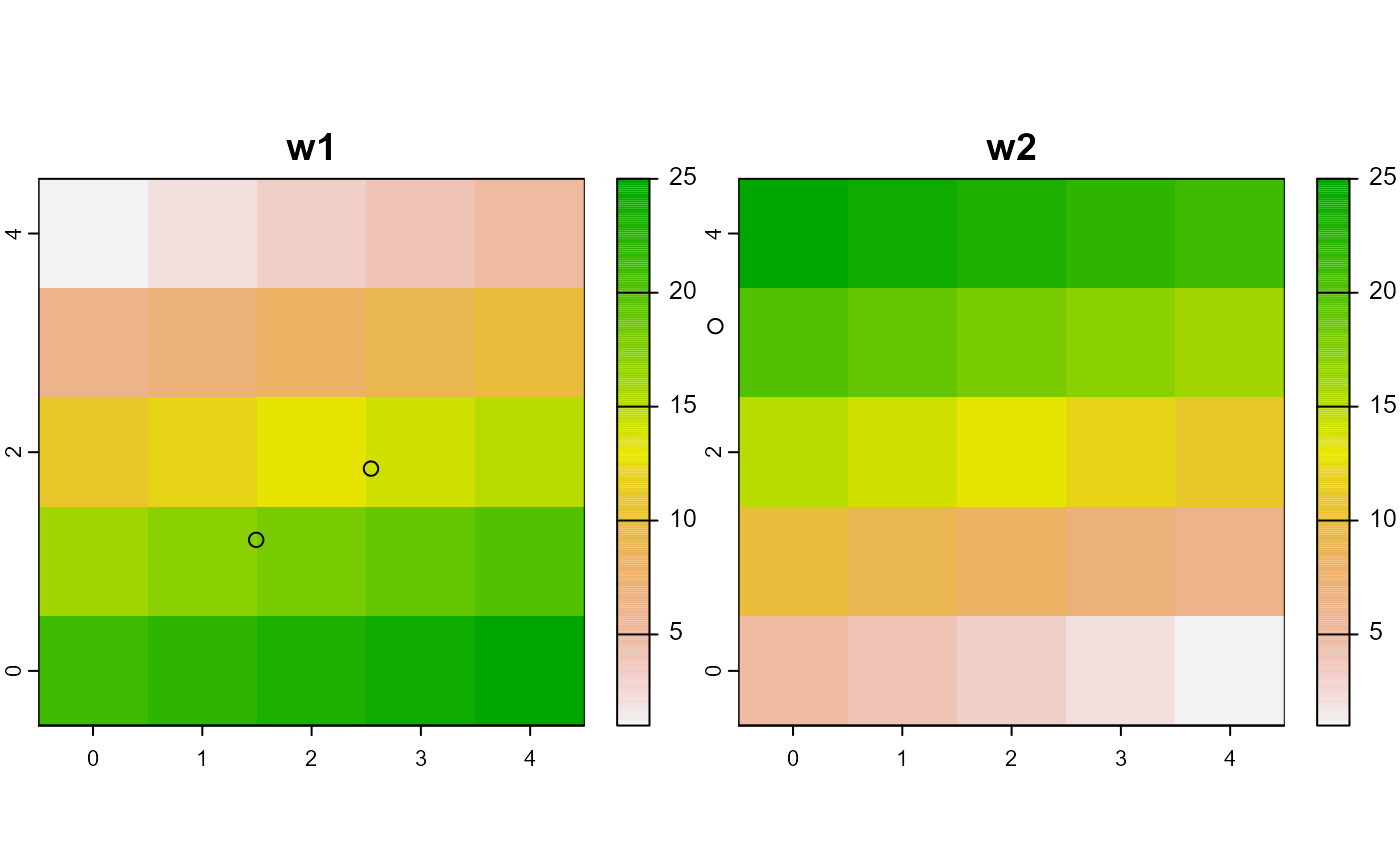These pass to plot, as a matrix of points (agentMatrix), as a raster (worldMatrix), or a rasterStack (worldArray). They can be modified.

# S3 method for agentMatrix
plot(x, ...)

# S3 method for worldMatrix
plot(x, ...)

# S3 method for worldArray
plot(x, ...)

# S3 method for agentMatrix
points(x, ...)

Arguments

x an agentMatrix, worldMatrix or worldArray object arguments passed to plot methods for matrix (agentMatrix) or raster (world*)

Value

none; invoked for side-effect of generating a plot.

Examples

# agentMatrix newAgent <- new("agentMatrix", coords = cbind(pxcor = c(1, 2, 5), pycor = c(3, 4, 6)), char = letters[c(1, 2, 6)], nums2 = c(4.5, 2.6, 2343), char2 = LETTERS[c(4, 24, 3)], nums = 5:7) plot(newAgent)## worldMatrix w1 <- createWorld(minPxcor = 0, maxPxcor = 9, minPycor = 0, maxPycor = 9, data = 1:100) plot(w1)## worldArray w1 <- createWorld(minPxcor = 0, maxPxcor = 4, minPycor = 0, maxPycor = 4, data = 1:25) w2 <- createWorld(minPxcor = 0, maxPxcor = 4, minPycor = 0, maxPycor = 4, data = 25:1) w3 <- stackWorlds(w1, w2) plot(w3)
# agentMatrix newAgent <- new("agentMatrix", coords = cbind(pxcor = c(1, 2, 5), pycor = c(3, 4, 6)), char = letters[c(1, 2, 6)], nums2 = c(4.5, 2.6, 2343), char2 = LETTERS[c(4, 24, 3)], nums = 5:7) points(newAgent)